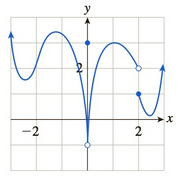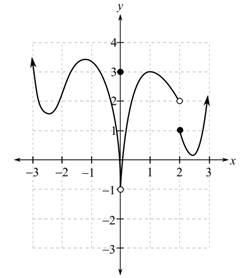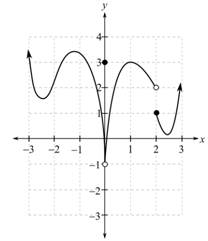Chapter 10, Problem 5REFinite Mathematics and Applied Cal...

7th Edition
Stefan Waner + 1 other
ISBN: 9781337274203

Solutions

Chapter
SectionFinite Mathematics and Applied Cal...

7th Edition
Stefan Waner + 1 other
ISBN: 9781337274203
Textbook Problem

In Exercises 5 and 6 the graph of a function f is shown. Graphically determine whether the given limits exist. If a limit does exist, give its approximate value.a. lim x → 0 f ( x ) b. lim x → 1 f ( x ) c. lim x → 2 f ( x )

(a)

To determine

The value of limx0f(x) if the limit exists using the graph which is as follows:Explanation

Given information:

The graph is as follows:

From the graph, determine the limit for x tends to 0 for the function f(x).

For this, take a point on the graph to the left of x=0 and move along the curve, so that the x-coordinate approaches 0. Then, it is noticed from the graph that f(x) appears to approach the open dot at the height of 1.

Similarly, take a point on the graph to the right of x=0 and when move along the curve to the left so that the x-coordinate approaches 0

(b)

To determine

The value of limx1f(x) if the limit exists using the graph which is as follows:(c)

To determine

The value of limx2f(x) if the limit exists using the graph which is as follows:Still sussing out bartleby?

Check out a sample textbook solution.

See a sample solution

The Solution to Your Study Problems

Bartleby provides explanations to thousands of textbook problems written by our experts, many with advanced degrees!

Get Started

Place the following set of n = 20 scores in a frequency distribution table.

Essentials of Statistics for The Behavioral Sciences (MindTap Course List)

Find the limit. lim0cos122

Calculus (MindTap Course List)

103/2

Applied Calculus for the Managerial, Life, and Social Sciences: A Brief Approach

Compute C5,2.

Understanding Basic Statistics

0 2 −2 does not exist

Study Guide for Stewart's Single Variable Calculus: Early Transcendentals, 8th

True or False: converges.

Study Guide for Stewart's Multivariable Calculus, 8th